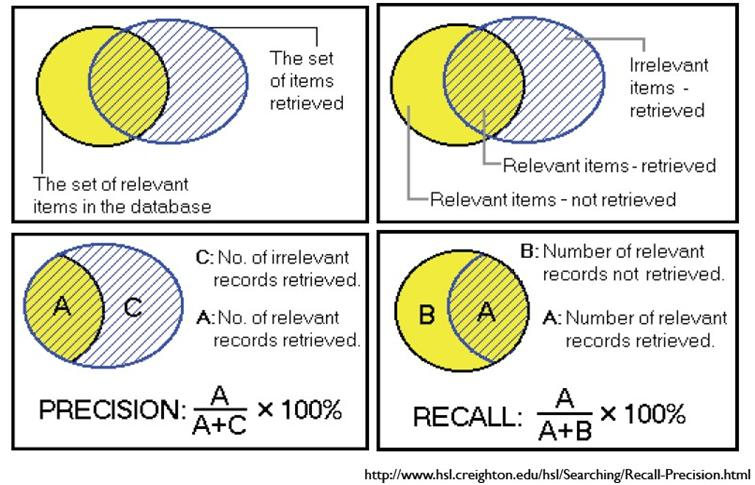# 准确率（Precision）、召回率（Recall）以及综合评价指标（F1-Measure ）Relevant NonRelevant Retrieved true positives （tp） false positives（fp） Not Retrieved false negatives（fn） true negatives （tn）

$P=\frac{tp} {tp+fp} \hfill (1)$

$R=\frac{tp} {tp+fn} \hfill (2)$

F1-Measure

F-Measure是Precision和Recall加权调和平均：

$F = \frac{(a^2+1)P*R} {a^2(P+R)} \hfill (3)$

$F1 = \frac{2PR} {P+R} \hfill (4)$

posted @ 2012-09-18 09:19  bluepoint2009  阅读(55902)  评论(1编辑  收藏  举报